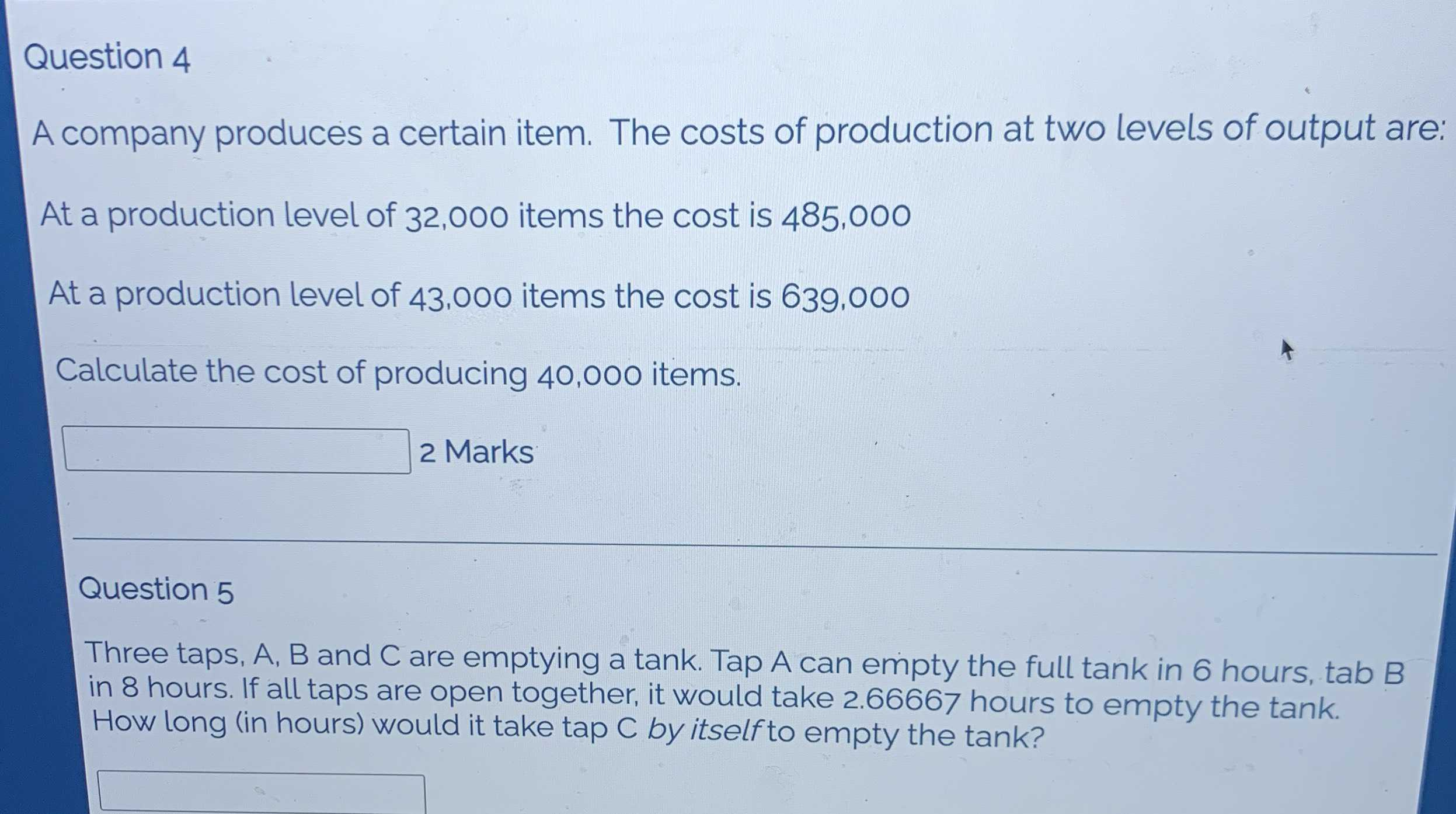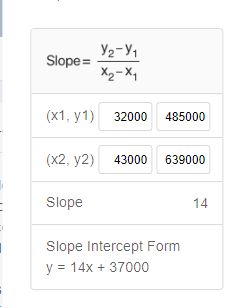### Still have math questions?

Algebra
QuestionQuestion $$4$$ A company produces a certain item. The costs of production at two levels of output are:

At a production level of $$32,000$$ items the cost is $$485,000$$

At a production level of $$43,000$$ items the cost is $$639,000$$

Calculate the cost of producing $$40,000$$ items.

Question $$5$$

Three taps, A, B and C are emptying a tank. Tap A can empty the full tank in $$6$$ hours, tab B in $$8$$ hours. If all taps are open together, it would take $$2.66667$$ hours to empty the tank. How long (in hours) would it take tap C by itself to empty the tank? Marks